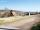# Two gardeners

The garden with an area of 81 square meters was divided by two gardeners in a ratio of 4: 5. How much did the second gardener get more first?

Result

x =  9 m2

#### Solution:Leave us a comment of example and its solution (i.e. if it is still somewhat unclear...):

Showing 0 comments:Be the first to comment!## Next similar examples:

1. GardenThe garden around the new majer is divided as follows: 35% vegetable, 30% fruit orchards, 10% flowers and the remaining 120 m2 are lawns. What is the total area of the garden?
2. CableCable consists of 8 strands, each strand consists of 12 wires with diameter d = 0.5 mm. Calculate the cross-section of the cable.
3. GlovesI have a box with two hundred pieces of gloves in total, split into ten parcels of twenty pieces, and I sell three parcels. What percent of the total amount I sold?
4. Trapezium 2Trapezium has an area of 24 square cms. How many different trapeziums can be formed ?
5. A trapezoidA trapezoid 75 ft wide on top 85 ft on the bottom, the height is 120 ft. What is its area in the square yds?
6. AcreagePlot has a diamond shape, its side is 25.6 m long and the distance of the opposite sides is 22.2 meters. Calculate its acreage.
7. In theIn the national park, the ratio of the wooded area to grassland is 4: 1. The total area is 385km2. What area is wooded?
8. RhombusPQRS is a rhombus. Given that PQ=3 cm & height of rhombus is given 2 cm. Calculate its area.
9. Pipe cross sectionThe pipe has an outside diameter 1100 mm and the pipe wall is 100 mm thick. Calculate the cross section of this pipe.
10. 1.5 divided1.5 divided by 1 = w divided by 4
11. Two numbersFind two numbers whose difference and ratio is 2.
12. DonutsFind how many donuts each student will receive if you share 126 donuts in a ratio of 1:5:8
13. Arble bagA marble bag sold by Rachel's Marble Company contains 5 orange marbles for every 6 green marbles. If a bag has 35 orange marbles, how many green marbles does it contain?
14. Percents - easyHow many percent is 432 out of 434?
15. Conference148 is the total number of employees. The conference was attended by 22 employees. How much is it in percent?
16. Simplify 2Simplify expression: 5ab-7+3ba-9
17. NumberWhat number is 20 % smaller than the number 198?